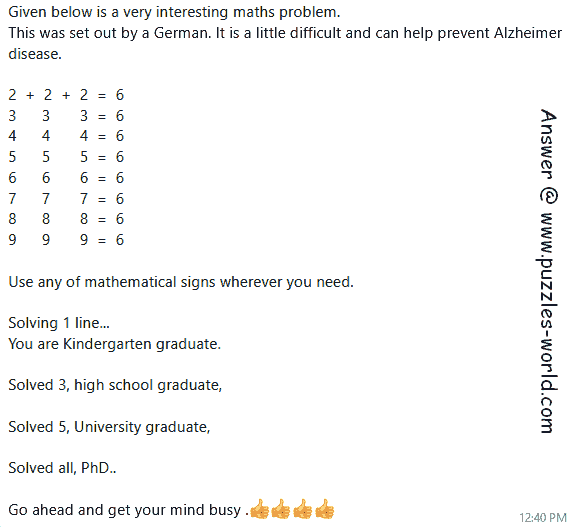# Use any mathematical signs 3 3 3 = 6## Use any mathematical signs to solve all the math problemsGiven below is a very interesting maths problem.
This was set out by a German.
It is a little difficult and
can help prevent Alzheimer disease.

Use any of mathematical signs wherever you need.
and solve all the math problems.
eg. 2 + 2 + 2 = 6

3 3 3 = 6
4 4 4 = 6
5 5 5 = 6
6 6 6 = 6
7 7 7 = 6
8 8 8 = 6
9 9 9 = 6

Use any of mathematical signs wherever you need.

Solving 1 line...

Solved all, PhD..